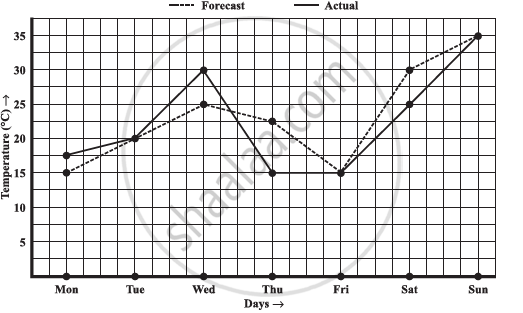# The Following Graph Shows the Temperature Forecast and the Actual Temperature for Each Day of a Week. - Mathematics

The following graph shows the temperature forecast and the actual temperature for each day of a week.1) On which days was the forecast temperature the same as the actual temperature?

2) What was the maximum forecast temperature during the week?

3) What was the minimum actual temperature during the week?

4) On which day did the actual temperature differ the most from the forecast temperature?

#### Solution

1) The forecast temperature was same as the actual temperature on Tuesday, Friday, and Sunday.

2) The maximum forecast temperature during the week was 35°C.

3) The minimum actual temperature during the week was 15°C.

4) The actual temperature differs the most from the forecast temperature on Thursday.

Concept: Concept of a Line Graph
Is there an error in this question or solution?

#### APPEARS IN

NCERT Class 8 Maths
Chapter 15 Introduction to Graphs
Exercise 15.1 | Q 4 | Page 238